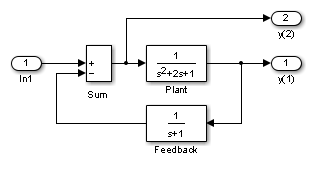## Linearizing Models

The Simulink® product provides the ``` linmod```, `linmod2`, and `dlinmod` functions to extract linear state-space models from Simulink models. However, these functions provide only basic linearization functionality. For full linearization functionality, use Simulink Control Design™ software. For more information, see Choose Linearization Tools (Simulink Control Design).

The `linmod`, `linmod2`, and `dlinmod` functions extract linear models in the form of the state-space matrices A, B, C, and D. State-space matrices describe the linear input-output relationship as

`$\begin{array}{l}\stackrel{˙}{x}=Ax+Bu\\ y=Cx+Du,\end{array}$`

where x, u, and y are state, input, and output vectors, respectively. For example, the following model is called `lmod`.To extract the linear model of this system, enter this command.

```[A,B,C,D] = linmod('lmod') A = -2 -1 -1 1 0 0 0 1 -1 B = 1 0 0 C = 0 1 0 0 0 -1 D = 0 1 ```

Inputs and outputs must be defined using Inport and Outport blocks from the Ports & Subsystems library. Source and sink blocks do not act as inputs and outputs. Inport blocks can be used in conjunction with source blocks, using a Sum block. Once the data is in the state-space form or converted to an LTI object, you can apply functions in the Control System Toolbox™ product for further analysis:

• Conversion to an LTI object

```sys = ss(A,B,C,D); ```
• Bode phase and magnitude frequency plot

```bode(A,B,C,D) or bode(sys) ```
• Linearized time response

```step(A,B,C,D) or step(sys) impulse(A,B,C,D) or impulse(sys) lsim(A,B,C,D,u,t) or lsim(sys,u,t) ```

You can use other functions in the Control System Toolbox and the Robust Control Toolbox™ products for linear control system design.

When the model is nonlinear, an operating point can be chosen at which to extract the linearized model. Extra arguments to `linmod` specify the operating point.

```[A,B,C,D] = linmod('sys', x, u) ```

For discrete systems or mixed continuous and discrete systems, use the function `dlinmod` for linearization. This function has the same calling syntax as `linmod` except that the second right-hand argument must contain a sample time at which to perform the linearization.